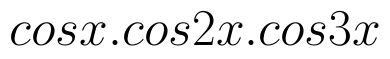Select Page

# MCQ Solutions for CBSE 12 Science Maths Application of Integrals in English

MCQ Solutions for CBSE 12 Science Maths Application of Integrals in English to enable students to get Solutions in a narrative video format for the specific question.

Expert Teacher provides MCQ Solutions for CBSE 12 Science Maths Application of Integrals through Video Solutions in English language. This video solution will be useful for students to understand how to write an answer in exam in order to score more marks. This teacher uses a narrative style for a question from Application of Integrals not only to explain the proper method of answering question, but deriving right answer too.

Please find the question below and view the Solution in a narrative video format.

Question:

Solution Video in English:

## Similar Questions from CBSE, 12th Science, Maths, Application of Integrals

Question 1 : Find the area of the region in the first quadrant enclosed by x-axis, lineand the circle(View Answer Video)

Question 2 : Given that, for x = 0 to x = 20. Find f(x) and g(x) such that the area between f(x) andfrom x = 0 to x = 5 beand area between g(x) andfrom y = 0 to y = 5 be. Is? Like functions f and g which work is better, team work or individual work? (View Answer Video)

Question 3 : Using integration, find the area bounded by the tangent to the curveat the point (2, 1) and the lines whose equations are x = 2y and x = 3y - 3. (View Answer Video)

Question 4 : Using the method of integration find the area bounded by the curve |x| + |y| = 1. (View Answer Video)

Question 5 : Using integration find the area of region bounded by the triangle whose vertices are (-1, 0), (1, 3) and (3, 2). (View Answer Video)

### Probability

Question 1 : Out of a group of 30 honest people, 20 always speak the truth. Two persons are selected at random from the group. Find the probability distribution of the number of selected persons who speak the truth. Also find the mean of the distribution. What values are described in this question?     (View Answer Video)

Question 2 : Three persons A, B and C apply for a  job of Manager in a Private company. Chance of their selection (A, B and C) are in the ratio 1:2:4. The probability that A, B, and C can introduce changes to improve profits of company are 0.8, 0.5 and 0.3 respectively, if the changes does not take place, find the probability that is due to the appointment of C.    (View Answer Video)

Question 3 : A coin is biased so that the heads is 2 times as likely to occur as tail. If the coin is tossed twice, find the probability distribution of number of tails. Hence find the mean of the distribution.  (View Answer Video)

Question 4 : A bag contains 4 red and 4 black balls, another bag contains 2 red and 6 black balls. One of the two bags is selected at random and two balls are drawn at random (without replacement) from the bag which are both found to be red. Find the probability that the balls are drawn from the first bag.    (View Answer Video)

Question 5 : A bag I contains 5 red and 4 white balls and a bag II contains 3 red and 3 white balls. Two balls are transferred from the bag I to the bag II and then one ball is drawn from bag II. If the ball drawn from the bag II is red, then find the probability that one red ball and one white ball are transferred from the bag I to the bag II.   (View Answer Video)

### Continuity and Differentiability

Question 1 : Findfor the function. (View Answer Video)

Question 2 : Differentiate the function w.r.t.x. (View Answer Video)

Question 3 : Findfor the function. (View Answer Video)

Question 4 : Differentiate the functionwith respect to x. (View Answer Video)

Question 5 : Differentiate the functionwith respect to x. (View Answer Video)

### Linear Programming

Question 1 : The objective function is maximum or minimum, which lies on the boundary of the feasible region. (View Answer Video)